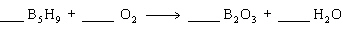Name:    Practice Test 3A

Multiple Choice

1.

Calculate the molar mass of (NH4)3AsO4
 A) 156.96 g/mol B) 165.02 g/mol C) 193.03 g/mol D) 417.80 g/mol

2.

Which of the following describes an oxidation?
 A) gain of electrons or gain of oxygen B) loss of electrons or gain of oxygen C) loss of electrons or gain of hydrogen D) loss of electrons or loss of oxygen

3.

Calculate the number of moles in 112 g of aspirin, C9H8O4.
 A) 0.619 B) 1.18 C) 0.165 D) 1.61

4.

In the reaction shown below, how many kcal of heat is required to produce 32.0 g of O2?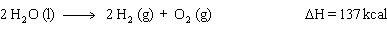A) 274 kcal B) 68.5 kcal C) 137 kcal D) 548 kcal

5.

How many atoms are present in 0.040 mol of O2?
 A) 2.4 x 1022 B) 4.8 x 1022 C) 6.6 x 1026 D) 1.5 x 1025

6.

Which of the following is an oxidation-reduction reaction?
 A) AgNO3  +  NaClAgCl  +  NaNO3 B) CaCl2  +  Na2(SO2)2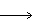CaSO2  +  2NaCl C) N2  +  O22NO D) KOH  +  HNO3H2O  +  KNO3

7.

Calculate the mass of 3.0 x 1022 atoms of sulfur.
 A) 0.16 g B) 1.6 g C) 160 g D) 16 g

8.

When the equation shown below is balanced, the proper sequence of coefficients for each substance is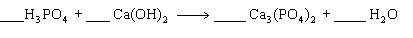A) 2, 3, 1, 6 B) 2, 3, 6, 1 C) 2, 3, 3, 1 D) 3, 2, 1, 6

9.

What is oxidized and what is reduced in the following reaction?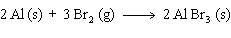A) Al is oxidized and Br2 is reduced. B) AlBr3 is reduced and Al is oxidized. C) Al is reduced and Br2 is oxidized. D) AlBr3 is reduced and Br2 is oxidized.

10.

The mineral Fluorite contains 51.5% by mass calcium.  How many grams of calcium are in a 135 g sample of Fluorite?
 A) 2.62 g B) 0.381 g C) 85.2 g D) 69.5 g

11.

The reaction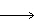is an example of
 A) decomposition B) combination C) single replacement D) double replacement

12.

What type of reaction is shown bleow?

CH4  +  2 O2CO2  +  2 H2O  +  218 kcal
 A) Exothermic B) Single replacement C) decomposition D) Endothermic

13.

In an endothermic reaction,
 A) the products have less energy than the reactants. B) energy is absorbed by the system. C) heat flow out of the system. D) temperature of the surroundings increases.

Short Answer

Balance each equation shown below:

14.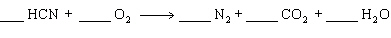15.